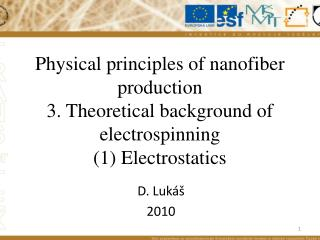# D. Lukáš 2010 - PowerPoint PPT PresentationDownload PresentationD. Lukáš 2010

D. Lukáš 2010Download Presentation## D. Lukáš 2010

- - - - - - - - - - - - - - - - - - - - - - - - - - - E N D - - - - - - - - - - - - - - - - - - - - - - - - - - -
##### Presentation Transcript

1. Physical principles of nanofiber production3. Theoretical background of electrospinning (1) Electrostatics D. Lukáš 2010

2. Literature: Feynman R P, Leighton R B, Sands M, Feynmans lectures from physics, Part 2, Fragment, Havlíčkův Brod, 2001. Chapter 4, Elektrostatics, str. 63 – 81 (=18 pages)

3. Electrospinningmay be thought to be a member of larger group of physical phenomena, classified as electrohydrodynamics. This important group of electrical appearances concerns the nature of ion distribution in a solution, caused by the influence of electric field, generated by organized groups of charges, to give a wide range of solution behaviour, such as, electrophoresis, electroosmosis, electrocapillarity and electrodiffusion, as recorded by Bak and Kauman . In this lecture will be briefly described how the theory of electrohydrodynamics has been evolving since the initial pioneering experimental observations. To start with, it is convenient to introduce an overview of the basic principles of electrostatics and capillarity to enable deeper understanding of physical principles of electrospinning.

4. Electrostatics Historically, the basic law of electrostatics is the Coulomb law, describing a force by which a charge acts on a charge on a distance in a space with electric permittivity, (3.1) y 1 2 x Feynmans lectures from physics, Part2, chapter 4.2

5. Coulomb force per unitary charge is called field strength or, field intensity and is commonly denoted as (3.2) y x

6. For electrostatic field, holds the superposition principle. For charges and that generate electrostatic fields with intensities and respectively, the resultant / joint field is determined by the following sum, (3.3) y 1 2 x

7. The space dependence of intensity generated by a point charge together with the superposition principle, leads to an alternative formulation of Coulomb law that is called Gauss Theorem of electrostatics. According to this theorem, the scalar product of intensity, E, with a surface area element ds, integrated along a closed surface S , is equal to a charge, q , trapped inside the close surface by permittivity, . The surface area element is considered here as a vector normal to the surface element. (3.4)

8. q S

9. Gauss’s principle in electrostatics describes electrostatic field property from macroscopic point of view. It has also a microscopic variant, given by: (3.5) This equation is also known as the First Maxwell Lawfor electrostatics. Hamilton Operator

10. z x y

11. Another consequence of Coulomb law is the fact, that electrostatic field is the conservative one and, hence, there exists a potential  that determines unequivocally the field intensity by means of the following relation z (3.6) x y

12. Maxwell equations for electrostatics

13. The substitution from the relation (3.6) into the equation (3.5) provide us with so-called Poisson Equation Laplace Equation Laplace Operator

14. Summary: • Coulomb law • Field Intensity • Superposition Principle • Gauss Theorem • First Maxwell Lawfor electrostatics • Hamilton Operator • Electrostatic field is the conservative one – potential • Poisson Equation and Laplace Equation • Laplace Operator

15. Homework: 1. Scalar product 2. 3. Show that pays: 4. Vector product